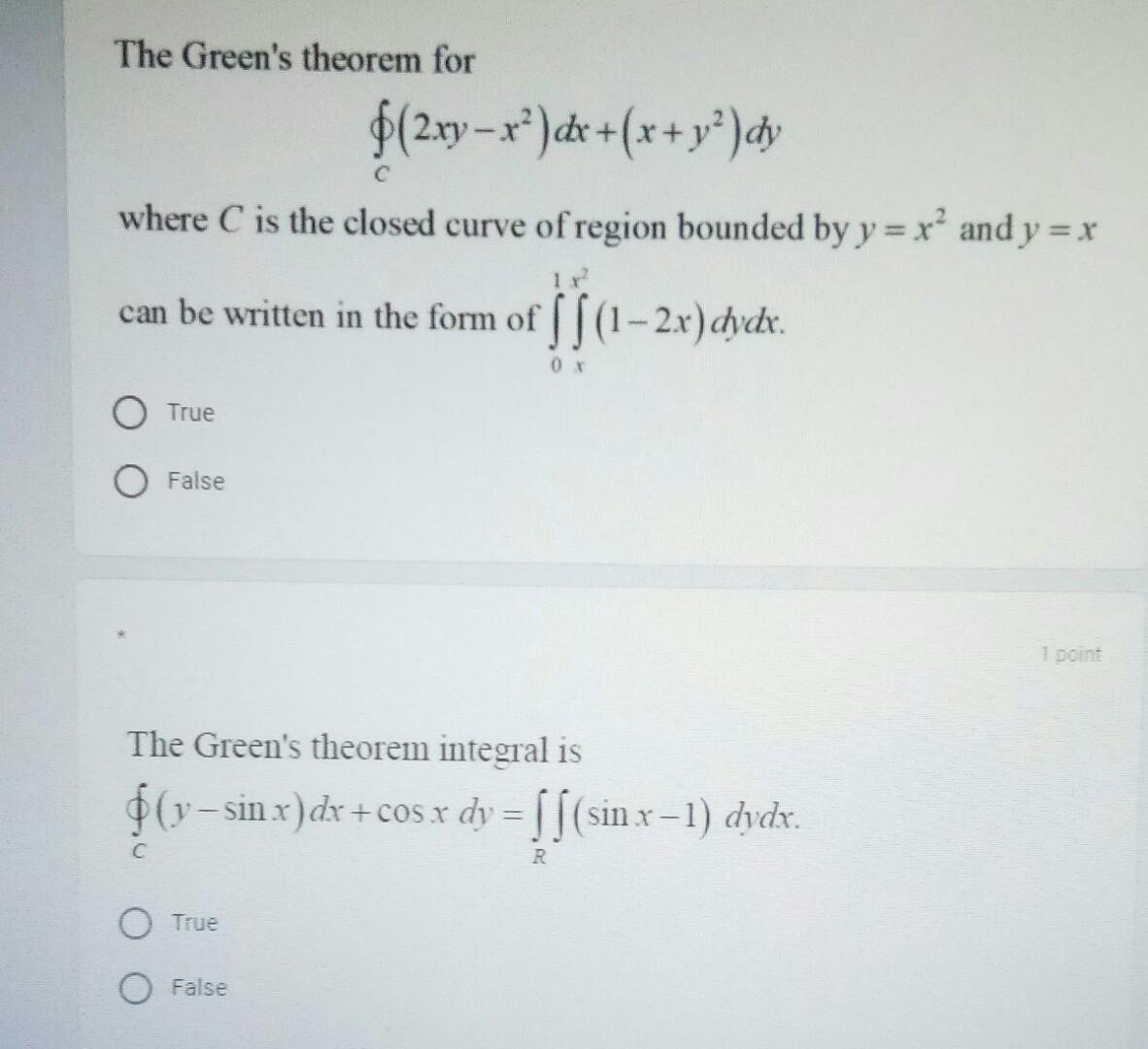### Create an Account

Already have account?

### Forgot Your Password ?

Home / Questions / The Green's theorem for \$(2xy = xº)dx+(x+y?)dy C where C is the closed curve of region bou...

# The Green's theorem for \$(2xy = xº)dx+(x+y?)dy C where C is the closed curve of region bounded by y = x? and y = x can be written in the form of ||(1-2x) dydx. bio 0 True O False 10 The Green's theore

The Green's theorem for \$(2xy = xº)dx+(x+y?)dy C where C is the closed curve of region bounded by y = x? and y = x can be written in the form of ||(1-2x) dydx. bio 0 True O False 10 The Green's theorem integral is \$(v- (y-sin x) dx + cos x dy = S/(sin x-1) dydx. C с R O True FalseApr 14 2021 View more View Less

#### Answer (Solved)Subscribe To Get Solution